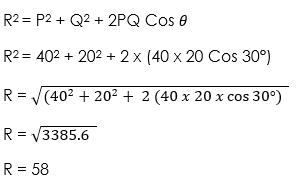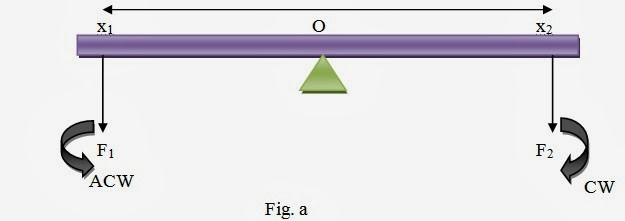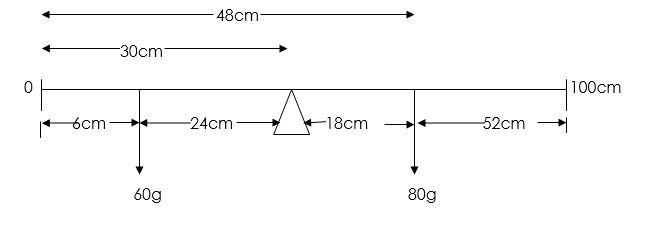# Equilibrium of Forces

Welcome to class!

In today’s class, we will be talking about the equilibrium of forces. Enjoy the class!

### Equilibrium of ForcesAn object is in equilibrium when it is not accelerated, that is there is no force acting on it in any direction. For a body in equilibrium, the forces acting on it are so related in magnitude and direction that no acceleration results.

A body is said to be in equilibrium when the body as a whole either remains at rest or moves in a straight line with constant speed and the body is either not rotating at all or is rotating at a constant angular velocity.

#### FORMS OF EQUILIBRIUM

1. Static equilibrium: This is when a body is at rest or moving with constant velocity.
2. Dynamic or Kinetic equilibrium: This is when a body is moving with a constant velocity in a straight line or rotating with a constant angular velocity about a fixed axis through its centre of mass.

#### RESULTANT AND EQUILIBRANT FORCES

A body acted upon by two or more forces is said to be in equilibrium if it does not move or rotate. Under this condition, the sum of the forces moving the body in one direction is equal to the sum of the forces pushing the body in the opposite direction. In the force board experiment, the resultant R of two forces P and Q acting at point O was found by the parallelogram method to be equal in magnitude but exactly opposite in direction to the third force that kept the point O in equilibrium. This third force is known as EQUILIBRANT FORCE.The Resultant force is that single force which acting alone will have the same effect in magnitude and direction as two or more forces acting together.

The Equilibrant of two or more forces is that single force which will balance all the other forces taken together. It is equal in magnitude but opposite in direction to the resultant force.

Couples and Torque

This is a pair of equal and parallel force acting on a body in opposite direction and whose lines of action do not coincide, which results In the direction of the body about its axis while Torque is the turning effect of a couple.

##### EQUILIBRIUM OF THREE FORCES ACTING AT A POINT

The three forces that keep a body in equilibrium can be represented in magnitude and direction by the three sides of a triangle as shown belowOB represents the force Q in magnitude and direction, OA represents the force P both in magnitude and direction and CO represents the equilibrium E in magnitude and direction. The triangle shown below shows below is the vector representation of the three forces acting at O.The principle of the triangle of forces states that if three forces are in equilibrium, they can be represented in both magnitude and direction by the three sides of a triangle taken in order.

MATHEMATICAL EXPRESSION

Resultant of two forces not at a right angle

By trigonometry for triangle ABC, we have that:

AB2 = BA2 + BD2 – 2AB x BD Cos AD

But Cos ABD = (180 – ) = – Cos θ

Therefore R2 = P2 + Q2 + 2PQ Cos θ

Where  = angle BAC, the angle between the forces P, Q = 180° – angle ADB.

Resultant of two forces at a right angle

The resultant of two forces at a right angle is given by:

R2 = AB2 + DB2

###### WORKED EXAMPLES

(1) The two forces of 20N and 40N, act at a point and angle between them is 30°. Find the resultant force.

SOLUTION

By trigonometry for triangle we have:

Resultant:Therefore the resultant force = 58N

The direction of the resultant force is obtained using sine rule:(2) if a force P of 40N acts in the direction due East and another force Q of 30N acts in the direction due North, calculate the resultant of these two forces.

SOLUTION

By Pythagoras theoremTherefore Resultant is 50N making 36.9° with 40N Force.

##### MOMENT OF A FORCE

When we turn on a tap, tighten a nut with a spanner or screw a nail in or out of wood with a screwdriver, we are exerting a turning force and producing a turning effect about a point along an axis. Such a turning effect brought about in each case is called MOMENT OF A FORCE.

Two factors are involved in each case:

1. The magnitude of force applied; and
2. The perpendicular distance of its line of action from the axis or pivot about which the turning effect is felt or exerted.

Therefore, the moment of a force about a point or axis is defined as the product of the force and the perpendicular distance of its line of action from the point.

Moment = force x perpendicular distance of the pivot to the line of action of the force. It is a vector quantity and its S.I. unit is Newton – meter (NM).

###### PRINCIPLE OF MOMENTS

The principle of moments states that if a body is in equilibrium then the sum of the clockwise turning moments acting upon it about any point equals the sum of the anticlockwise turning moments about the same point.From fig. a above, clockwise moment about O is given as F2x2 while the anticlockwise moment is F1x1. Therefore, the sum of the moment is given as F2x2 – F1x1. If the bar is in equilibrium,

F2x2 – F1x1 = 0

F2x2 = F1x1.

In fig.b, taking moment about O,

F1x1 – F2x2 + F3x3 – F4x4.

If the bar is in equilibrium,

F1x1 – F2x2 + F3x3 – F4x4 = 0

F1x1 + F3x= F2x2 + F4x4.##### CENTER OF GRAVITY

The centre of gravity of a body is defined as the point through which its resultant weight acts.

###### CONDITIONS FOR EQUILIBRIUM OF NON – PARALLEL FORCES
• For the action of parallel coplanar forces
1. The algebraic sum of the forces acting on the body in any direction must be zero. That is the Sum of upward forces = Sum of downward forces.
2. The algebraic sum of the moments acting on the body in any direction must be zero. That is the Sum of CW moment = Sum of ACW moment.
3. The total resultant force must be zero
• For the action of non – parallel coplanar forces
1. The vector sum of all the forces acting on the body must be zero. That is, Σx = 0 and Σy = 0.
2. The algebraic sum of the moments acting on the body in any direction must be zero. That is the Sum of CW moment = Sum of ACW moment.
3. The line of action of the forces must meet at a point
###### WORKED EXAMPLE

(1) A metre rule is found to balance horizontally at the 48cm mark. When a body of mass 60g is suspended at the 6cm mark, the balance point is found to be the 30cm mark.

Calculate the:

(i) Mass of the metre rule

(ii) Distance of the balance point from the zero end of the body was moved to the 13cm mark.

SOLUTIONLet the mass of the rule be represented by w.Let the distance of the new balance point be x from zero endIn our next class, we will be talking about the Equilibrium of Bodies in Liquid.  We hope you enjoyed the class.

Should you have any further question, feel free to ask in the comment section below and trust us to respond as soon as possible.

How Can We Make ClassNotesNG Better - CLICK to Tell Us💃

### 4 thoughts on “Equilibrium of Forces”

1.princesscharles109

Please how could we know how to represent the three magnitude and direction in the triangle

2.I so much love this class
Nice one

3.Please why the 3 and 4 in the last solving

4.What is the formula for Mass of meter rule,and the distance from the balance point.

Don`t copy text!